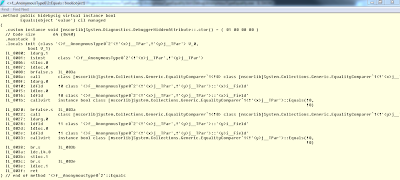# Jef Claes

On software and life

After publishing yesterday’s post on anonymous type equality, I received an interesting comment. The comment stated that even if the sequence of the property assignment were the same, the equality comparison would still return false, because the types generated by the C# compiler are reference types, making their references being tested for equality and not their data.

This is very true, unless the `Equals()` method is overridden. And this is exactly what the compiler does for us when we define anonymous types.In the `Equals()` method the equality of each property is evaluated by using a generic EqualityComparer.

That explains this behaviour.

``````var a = new { x = 1, y = 2 };
var b = new { y = 2, x = 1 };
var c = new { x = 1, y = 2 };

Console.WriteLine(a.Equals(b)); //False
Console.WriteLine(a.Equals(c)); //True
``````